×

Search anything:

# Priority Queue in C++ STL

#### C++ Software Engineering standard template library priority queueThe priority queue in the STL of C++ is a dynamically resizing container to implement the special case of priority queye under the queue data structure. It is a sequential linear container. The top element of the priority queue is the greatest with all elements arranged in non-increasing order.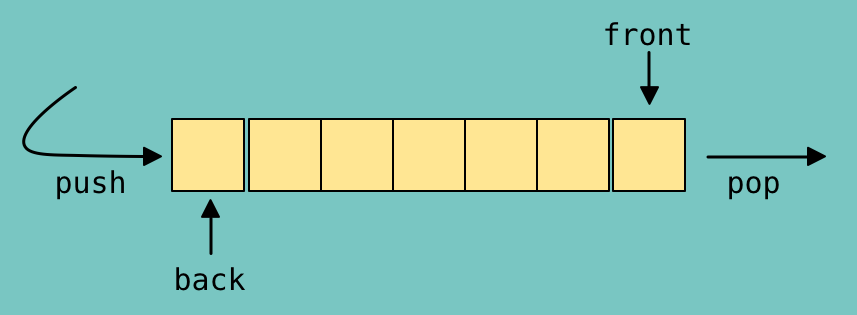### Definition in `<queue>`

std::priority_queue is defined as follows under `<queue>` header file:

``````template <class T, class Container = vector<T>, class Compare = less<typename Container::value_type> > class priority_queue;
``````

### Declaration

A priority queue with name a storing integers is declared as follows:

``````priority_queue<int> a;
``````

### Functions

Assume the priority queue a to be: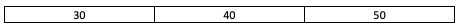and the priority queue b to be: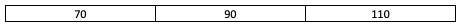The following functions are defined under the `<queue>` header file:

###### Note
• The worst case time complexity of inserting an element using emplace/push is `O(NlogN)` as the elements are reordered according to priority after insertion.
• Removing an element using pop operation is an `O(1)` operation as the element at the top is immediately removed.

### Illustration of Queue

``````// C++ code to illustrate priority queue in Standard Template Library (STL)
#include <iostream>
#include <queue>

using namespace std;

void display(priority_queue <int> a)
{
priority_queue <int> c = a;
while (!c.empty())
{
cout << '\t' << c.top();
c.pop();
}
cout << '\n';
}

int main ()
{
priority_queue <int> a;
a.push(10);
a.push(30);
a.push(20);
a.push(5);
a.push(1);

cout << "The priority queue a is : ";
display(a);

cout << "a.size() : \n" << a.size();
cout << "a.top() : \n" << a.top();

cout << "a.pop() : \n";
a.pop();
display(a);

return 0;
}
``````
``````\\Console output
The priority queue a is :     30    20    10    5    1
a.size() : 5
a.top() : 30
a.pop() :     20    10    5    1
``````
###### State of Queue a after each iteration: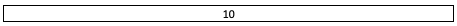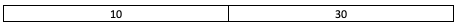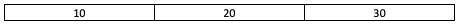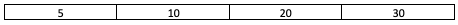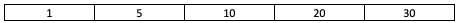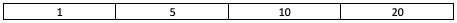### Question

#### Choose the correct option

The top element in priority queue is the least
The top element in priority queue is the greatest
Priority Queue is a container which is dynamically arranged in a non-increasing order starting from the top whenever a push/pop operation is performed.